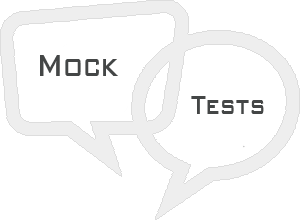# SVG - Mock Test

This section presents you various set of Mock Tests related to SVG Framework. You can download these sample mock tests at your local machine and solve offline at your convenience. Every mock test is supplied with a mock test key to let you verify the final score and grade yourself.# SVG Mock Test IV

Q 1 - Size can be big even for small SVG image.

A - true

B - false

### Explanation

Size can be big even for small SVG image.

Q 2 - What is the default value of x-axis co-ordinate of top left of the rectangle?

A - 0

B - -1

C - Any arbitrary value.

D - None of the above.

### Explanation

Default value is 0.

Q 3 - What is the default value of y-axis co-ordinate of top left of the rectangle?

A - 0

B - -1

C - Any arbitrary value.

D - None of the above.

### Explanation

Default value is 0.

Q 4 - What is the default value of x-axis co-ordinate of center of the circle?

A - 0

B - -1

C - Any arbitrary value.

D - None of the above.

### Explanation

Default value is 0.

Q 5 - What is the default value of y-axis co-ordinate of center of the circle?

A - 0

B - -1

C - Any arbitrary value.

D - None of the above.

### Explanation

Default value is 0.

Q 6 - What is the default value of x-axis co-ordinate of center of the ellipse?

A - 0

B - -1

C - Any arbitrary value.

D - None of the above.

### Explanation

Default value is 0.

Q 7 - What is the default value of y-axis co-ordinate of center of the ellipse?

A - 0

B - -1

C - Any arbitrary value.

D - None of the above.

### Explanation

Default value is 0.

Q 8 - What is the default value of x-axis co-ordinate of start point of the line?

A - -1

B - 0

C - Any arbitrary value.

D - None of the above.

### Explanation

Default value is 0.

Q 9 - What is the default value of y-axis co-ordinate of start point of the line?

A - -1

B - 0

C - Any arbitrary value.

D - None of the above.

### Explanation

Default value is 0.

Q 10 - What is the default value of x-axis co-ordinate of end point of the line?

A - -1

B - 0

C - Any arbitrary value.

D - None of the above.

### Explanation

Default value is 0.

Q 11 - What is the default value of y-axis co-ordinate of end point of the line?

A - -1

B - 0

C - Any arbitrary value.

D - None of the above.

### Explanation

Default value is 0.

Q 12 - Which of the following is used to pass points to draw a polygon?

A - points

B - d

C - path

D - None of the above.

### Explanation

points − List of points to make up a polygon.

Q 13 - Which of the following is used to pass points to draw a polyline?

A - points

B - d

B - path

B - None of the above.

### Explanation

points − List of points to make up a polyline.

Q 14 - Which of the following is used to pass points to draw a free flow path?

A - points

B - d

C - path

D - None of the above.

### Explanation

d − path data,usually a set of commands like moveto, lineto etc.

Q 15 - m command of path element move from one point to another point.

A - true

B - false

### Explanation

m command of path element move from one point to another point.

Q 16 - l command of path element move from one point to another point.

A - true

B - false

### Explanation

m command of path element move from one point to another point.

Q 17 - l command of path element creates a line.

A - true

B - false

### Explanation

l command of path element creates a line.

Q 18 - h command of path element creates a vertical line.

A - true

B - false

### Explanation

h command of path element creates a horizontal line.

Q 19 - h command of path element creates a horizontal line.

A - true

B - false

### Explanation

h command of path element creates a horizontal line.

Q 20 - v command of path element creates a vertical line.

A - true

B - false

### Explanation

v command of path element creates a vertical line.

Q 21 - v command of path element creates a horizontal line.

A - true

B - false

### Explanation

v command of path element creates a vertical line.

Q 22 - c command of path element creates a curve.

A - true

B - false

### Explanation

c command of path element creates a curve.

Q 23 - s command of path element creates a smooth curve.

A - true

B - false

### Explanation

s command of path element creates a smooth curve.

Q 24 - q command of path element creates a quadratic bezier curve.

A - false

B - true

### Explanation

q command of path element creates a quadratic bezier curve.

Q 25 - t command of path element creates a smooth quadratic bezier curve.

A - false

B - true

### Explanation

q command of path element creates a smooth quadratic bezier curve.

1 A
2 A
3 A
4 A
5 A
6 A
7 A
8 B
9 B
10 B
11 B
12 A
13 A
14 B
15 A
16 B
17 A
18 B
19 A
20 A
21 B
22 A
23 A
24 B
25 B